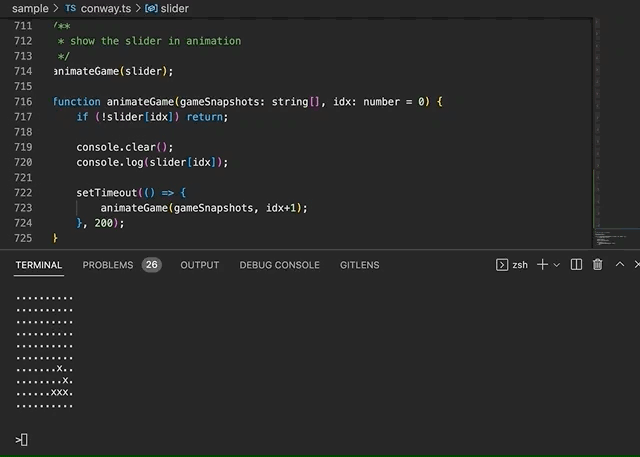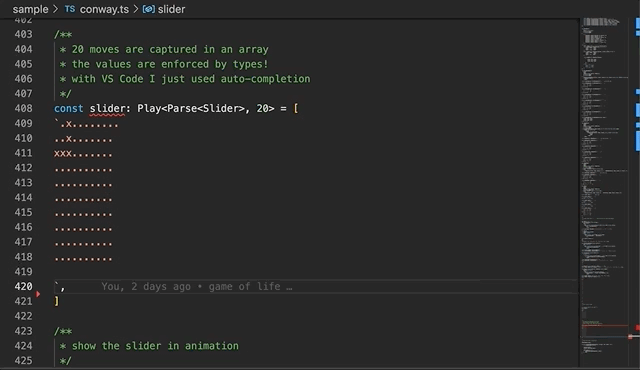Hackle's blog
between the abstractions we want and the abstractions we get.

# Is TYPEScript Turing complete? Not sure. Game of life? Why not!You would have recognised this "slider" as in Conway's game of life. Unlike others, this one is written in TYPEScript. Not TypeScript, as... it's written in TYPES. Mostly so.

To clarify, types alone do not make up an executable program, but they can constrain the values in a program, in this case, the state of the game is tightly controlled by the types. How tight? To the point that VS Code auto completes the values.As you can see the job left for "value-level" code is trivial, because "type-level" code dictates values.

This is where people would claim "TYPEScript type system is turing-complete"! However, let's not obsess over such claims. What's more interesting is how is this made possible?

It would be obvious but please do note this is not something you would put in production. Maybe for pair programming at an interview :-)

From this point on it would be more fun if you load up the code in VS Code (or your favourite IDE).

## Peano numbers again

With the introduction of conditional types (ages ago), it was already possible to have conditional or recursive type level computation. A big blocker for me to make the game of life has been numbers and ways to manipulate numbers. For example, I must be able to add or subtract numbers to find neighbouring cells for a cell at a coordinate.

I showed in a previous post how to represent Peano numbers in types:

``````type Nat = 0 | { suc: Nat };
``````

The problem with Peano numbers is they don't translate to arabic numbers very well (feel free to try and definitely let me know how that goes), whereas the latter is what we use for values. Recently I came to know of a breakthrough, or arguably also a hack, utilizing tuple/array. It goes as:

• a tuples is an array, so we can get its `length` (a number!), e.g. `const len: [number, string]['length'] = 2`
• we can also check the length of a tuple with `extends`, e.g. `const lenIs2: [number, string]['length'] extends 2 ? true : false = true`
• we can encode numbers as tuples by starting with an empty tuple, then keep appending elements to it until the length is filled, e.g.
``````type Peano<N extends number, Aggr extends any[] = []> =
Aggr['length'] extends N ? Aggr : Peano<N, [1, ...Aggr]>;
``````
• conversely, we can add elements to, or take elements away from a tuple to simulate addition and subtraction. While it's possible via iterations, it's done more efficiently through pattern matching.
``````type Minus<N1 extends number, N2 extends number> =
Peano<N1> extends Peano<N2>
? 0
: Peano<N1> extends [...Peano<N2>, ...infer Rest]
? Rest['length']
: never;

type Plus<N1 extends number, N2 extends number> =
[...Peano<N1>, ...Peano<N2>]['length'] extends infer R
? R extends number
? R : never
: never;
``````
• comparing numbers can be done similarly,
``````type Lte<N1 extends number, N2 extends number> = Peano<N2> extends [...Peano<N1>, ...infer _] ? true : false;
type Gte<N1 extends number, N2 extends number> = Peano<N1> extends [...Peano<N2>, ...infer _] ? true : false;
``````

## Game rules

With numbers sorted it's straightforward to implement the logic of the game. For example, to decide if a cell should live or die.

``````type MapOneCell<
Alive,
R extends number,
C extends number,
> = AliveNeighbours<Alive, Dead, Coords, R, C> extends infer Nc extends number
? Eq<Nc, 3> extends true
? Alive
: Eq<Nc, 2> extends true
? Eq<ValueAt<(Alive | Dead), Coords, R, C>, Alive> extends true
? Alive

const mapOneCell1: MapOneCell<'x', ' ', [['x']], 0, 0> = ' ';

const mapOneCell2: MapOneCell<'x', ' ', [
['x', 'x', 'x'],
['x', 'x', 'x'],
['x', 'x', 'x']
], 0, 0> = 'x';
``````

Though rigorous, type-leveling programming can also be a bit tedious. There is a lot of `extends` as it is used both for pattern matching and control flow (including recursion). Thankfully TypeScript 4.7.1 made it less verbose by allowing extends Constraints on infer Type Variables.

## Build from small and testable types

Coding with types may sound very different to that with values, but best practices still apply. For one, it's best to keep types small, so testing is easy; as always, values are tests for types.

These are the types from small to big for the game.

``````// count live cells
type CountAlive<Xs extends unknown[], Alive>

// find a value by coordinates in an matrix
type ValueAt<T, Coords extends T[][],
R extends number | never,
C extends number | never,
>

// find all neighbouring cells
type Neighbours<
T,
Coords extends T[][],
R extends number,
C extends number
>

// count live neighouring cells (combining some of the types above)
type AliveNeighbours<
Alive,
R extends number,
C extends number
>

// map one cell by coordinates
type MapOneCell<
Alive,
R extends number,
C extends number,
>

// map one row of cells
type MapOneRow<
Alive,
R extends number,
C extends number = 0,
>

// next state of a game (matrix)
type Next<
Alive,
R extends number = 0
>
``````

If you trace through these types, each will be followed by a few values of this type.

## The game runner

A few utility types are made to run the game.

``````// parse the initial state of a game (cells) from a string with 'x' and '.'
type ParseRow<Row extends string>
type Parse<Input = string>

// serialise cells for display
type SerializeRow<Row extends Cell[]>
type Serialize<T extends Cell[][]>

// play a game with initial state and number of moves
type Play<T extends Cell[][], Moves extends number>

const play1: Play<typeof state1, 5> = [...auto complete here...];
``````

Before this point, no values are required, it's all type-level stuff. Eventually, the types result in values. The value `const moves = Play<'x..x..', 5>` will contain 5 consecutive moves, calculated by the `Play` type. We then lapse into value-level programming, i.z. a function written in vanilla TypeScript to loop through these 5 moves for animated display.

That's it. It's not something that I should be doing every day, but it's definitely fun. Go grab the code here and have a play!